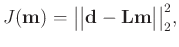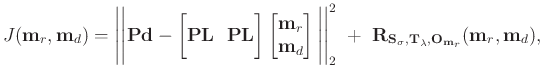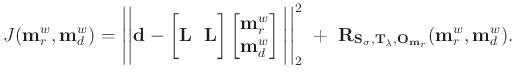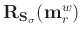## Objective function

Seismic imaging can be formulated as an optimization problem (Nemeth et al., 1999; Ronen and Liner, 2000):(5)

wherecorresponds to the modeling operator,corresponds to seismic data,is an image of the subsurface containing both reflections and diffractions. In this work we use Kirchhoff modeling and migration as forward () and adjoint () operators correspondingly. To image reflections and diffractions as separate components of a wavefield we propose the following objective function:(6)

Here,is forward Kirchhoff modeling operator,andare diffraction and reflection images (models) respectively,stands for analytical path-summation migration operator computed from equation 2 (corresponds toincluding forward Fourier, inverse Fourier andtransforms),is a plane-wave destruction filter applied in the data domain. To penalize the objective function and perform reflection/diffraction separation the shaping regularization operatoris designed (see the “Regularization” subsection for details).

Rather than fitting seismic dataas in equation 5, we search for models minimizing a misfit weighted by path-summation migration operator. Path-summation operatorpreceded by plane-wave destruction filter applied in the data domain () for reflection energy elimination can be treated as diffraction probability at a certain location. Therefore, model fitting becomes constrained to those samples, in which diffractions are most probable. The terms of the misfit in equation 6 can be rearranged to stress the misfit weighting by diffraction probability ():(7)

Incorporation of PWD () as a misfit weight into the inversion (equation 6) puts some of the reflected energy in the null space. PWD is a crucial part of the inversion. By weighting the residual with path-summation integral and PWD we guide the inversion to extract diffractions from the full wavefield, which is predominated by reflections. To restore reflections from the null space and account for their leakage to the diffraction image domain PWD can be disabled and another inversion cascade can be run:(8)

whereis the shaping regularization operator designed to perform reflection/diffraction separation after PWD operator () deactivation (see “Regularization” subsection for details).

Path-summation integral acts as a dip filter suppressing events with high wavenumbers (Merzlikin and Fomel, 2017a). High wavenumbers can be restored by running the third cascade of the inversion with path-summation integral filter disabled:(9)

A practical alternative is to restore high wavenumbers separately for reflections and diffractions. Once reflectionand diffractioncomponents are restored (after the second inversion cascade 8), they can be used as starting models in the following inversions for wavenumber restoration:(10)

whereare reflections with high wavenumbers restored, which are initialized withat the iteration zero. High wavenumbers in diffractionscan be restored as(11)

Reflections restored by equation 10 () are subtracted from the full wavefield datato allow for diffraction-only restoration. No interference is expected between reflectionsand diffractionsprovided accurate starting models from the previous inversions (equations 6 and 8).

Instead of implementing,,andas penalty terms for reflections and diffractions correspondingly, the problem is reformulated in the shaping framework (see “Regularization” subsection for details).

2019-07-17